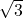## An acute angle of a right triangle measures 30°, and the length of the triangle’s hypotenuse is 10 ft. Find the missing angle measure and si

Question

An acute angle of a right triangle measures 30°, and the length of the triangle’s hypotenuse is 10 ft. Find the missing angle measure and side lengths.

in progress 0
2 months 2021-07-22T16:43:25+00:00 1 Answers 5 views 0

missing angle <60 and side lengths 5, 5Step-by-step explanation:

we understand from the given information that  the triangle in question is a special right triangle

and since this is a special triangle the side lengths follows :

the side length that sees <90 is represented by 2x

the side length that sees <60 is represented by xand the side length that sees <30 is represented by x

2x = 10 so x = 5 and  x= 5